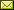## On the Lambek Calculus with an Exchange Modality

 Jiaming Jiang (North Carolina State University) Harley Eades III (Augusta University) Valeria de Paiva (Nuance Communications)

 In this paper we introduce Commutative/Non-Commutative Logic (CNC logic) and two categorical models for CNC logic. This work abstracts Benton's Linear/Non-Linear Logic by removing the existence of the exchange structural rule. One should view this logic as composed of two logics; one sitting to the left of the other. On the left, there is intuitionistic linear logic, and on the right is a mixed commutative/non-commutative formalization of the Lambek calculus. Then both of these logics are connected via a pair of monoidal adjoint functors. An exchange modality is then derivable within the logic using the adjunction between both sides. Thus, the adjoint functors allow one to pull the exchange structural rule from the left side to the right side. We then give a categorical model in terms of a monoidal adjunction, and then a concrete model in terms of dialectica Lambek spaces.

In Thomas Ehrhard, Maribel Fernández, Valeria de Paiva and Lorenzo Tortora de Falco: Proceedings Joint International Workshop on Linearity & Trends in Linear Logic and Applications (Linearity-TLLA 2018), Oxford, UK, 7-8 July 2018, Electronic Proceedings in Theoretical Computer Science 292, pp. 43–89.
Published: 15th April 2019.

 ArXived at: https://dx.doi.org/10.4204/EPTCS.292.4 bibtex PDF

 Comments and questions to:eptcs@eptcs.org For website issues:webmaster@eptcs.org